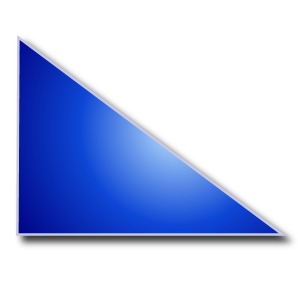Games
Problems
Go Pro!

# Coordinate Geometry Problem with Four Solutions

Lesson Plans > Mathematics > Geometry > Analytic Geometry > Linear

## Coordinate Geometry Problem with Four SolutionsYesterday in my pre-calculus class, I introduced the problem below, and asked my students to work on their own trying to solve it. The problem was unlike anything I'd done with them in the past, but they had learned everything they needed to know in order to solve it in at least a couple ways. I was curious to see if they would put the pieces together. Here's the problem (which they all solved, with varying degrees of hints and suggestions):

The lines y =
3
4
x, y =
4
3
x, and y = -x + 7 bound a polygon. What is the area of that polygon?

All the students began by graphing the lines, and discovering that they bounded a triangle, and that the vertices were at (0,0), (3,4), and (4,3). From here they went in several directions. By the time class was over, we had discussed four different ways of solving the problem.

Method One
1. Find the length of the segment from (3,4) to (4,3).
2. Find the midpoint of that segment.
3. Find the length of the segment from (0,0) to (the midpoint of (3,4) and (4,3)
4. The two segments we've found the length of are base and height, so we can use 1/2bh to calculate the area.

Method Two
1. Find the lengths of the 3 sides of the triangle.
2. Use Heron's Fomula (A =s(s - a)(s - b)(s - c)
, where s is the semiperimeter) to find the area.

Method Three
1. Draw a 4 x 4 square around the triangle.
2. Calculate the areas of the three right triangles that surround our triangle.
3. Subtract these areas from the area of the square.

Method Four
1. Use trigonometry to calculate the angle of inclination of the two lines y =
3
4
x and y =
4
3
x.
2. Subtract these to obtain the angle between the lines.
3. Calculate the lengths of the two sides adjacent to this angle.
4. Use A =
1
2
absinC to find the area.

Each solution produces an answer of 3.5 square units.

Conclusion
This problem gave me the opportunity to introduce two new formulas (the formulas in methods 2 and 4), plus it allowed the students to see that there are often multiple ways to solve the same problem, and if they can't get it one way, that doesn't mean they should give up!

Lesson by Mr. Twitchell# Blogs on This SiteReviews and book lists - books we love!The site administrator fields questions from visitors.Like us on Facebook to get updates about new resources